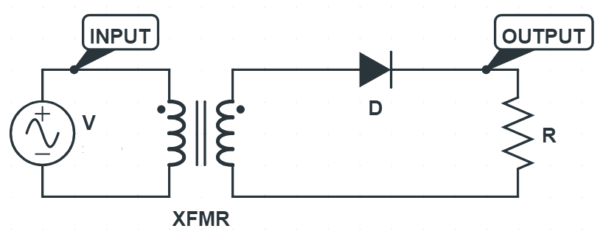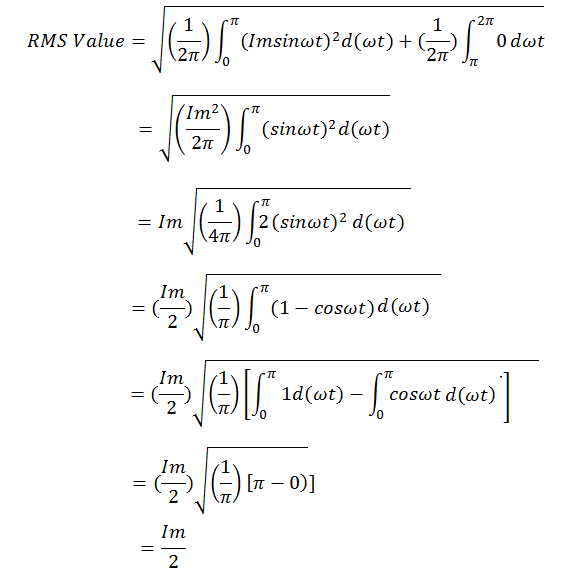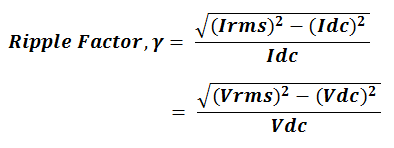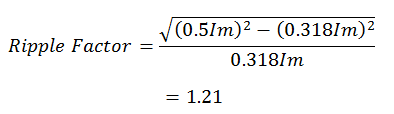# Ripple Factor of Half Wave Rectifier

The value of ripple factor of single phase half wave rectifier is equal to 1.21. Since, ripple factor is the ratio of rms value of fluctuating ac component to the average value or dc value. Its value of 1.21 means that, the ac fluctuating component in the rectified output of half wave rectifier is 121% of the expected DC output or average vale. This simply means, the rectification is poor as the ripple content is even higher than the DC output. Let us now see the calculation of this ripple factor.

Calculation of Ripple Factor of Half Wave Rectifier

The circuit diagram of single phase half wave rectifier is shown below.The diode will be forward biased for the positive half cycle of the supply whereas it will be reverse biased for the negative half cycle. Thus the diode will conduct only for the positive half cycle and will not conduct for the negative half cycle. Therefore, current output will only be conducting and allowing the current to flow just for the positive half cycle of the supply. This is the reason; it is called single phase half wave rectifier.

The output waveform of half wave rectifier ignoring cut-in voltage is shown in figure below. Blue color depicts the supply voltage whereas red color denotes the rectifier output current.This output current can be defined as

i = Imsinωt for 0≤ωt≤π

= 0 for π≤ωt≤2π

Let us now find the rms value and average value of this current to find the ripple factor of half wave rectifier.Thus, the rms value of current output of half wave rectifier is 0.5 times of the peak current i.e. 0.5Im.

The average value of single phase half wave rectifier is equal to 0.318 times of peak current i.e. 0.318Im. For derivation, please read “Average Value of AC Current – Definition, Formula and Application

Now, the ripple factor of half wave rectifier can easily be calculated from the formula.Putting the value of rms current and average current, the value of ripple factor can easily be calculated from the above formula.Thus, the value of ripple factor of half wave rectifier is 1.21.

### One thought on “Ripple Factor of Half Wave Rectifier”

1.Hadj says:

thank you
T

This site uses Akismet to reduce spam. Learn how your comment data is processed.